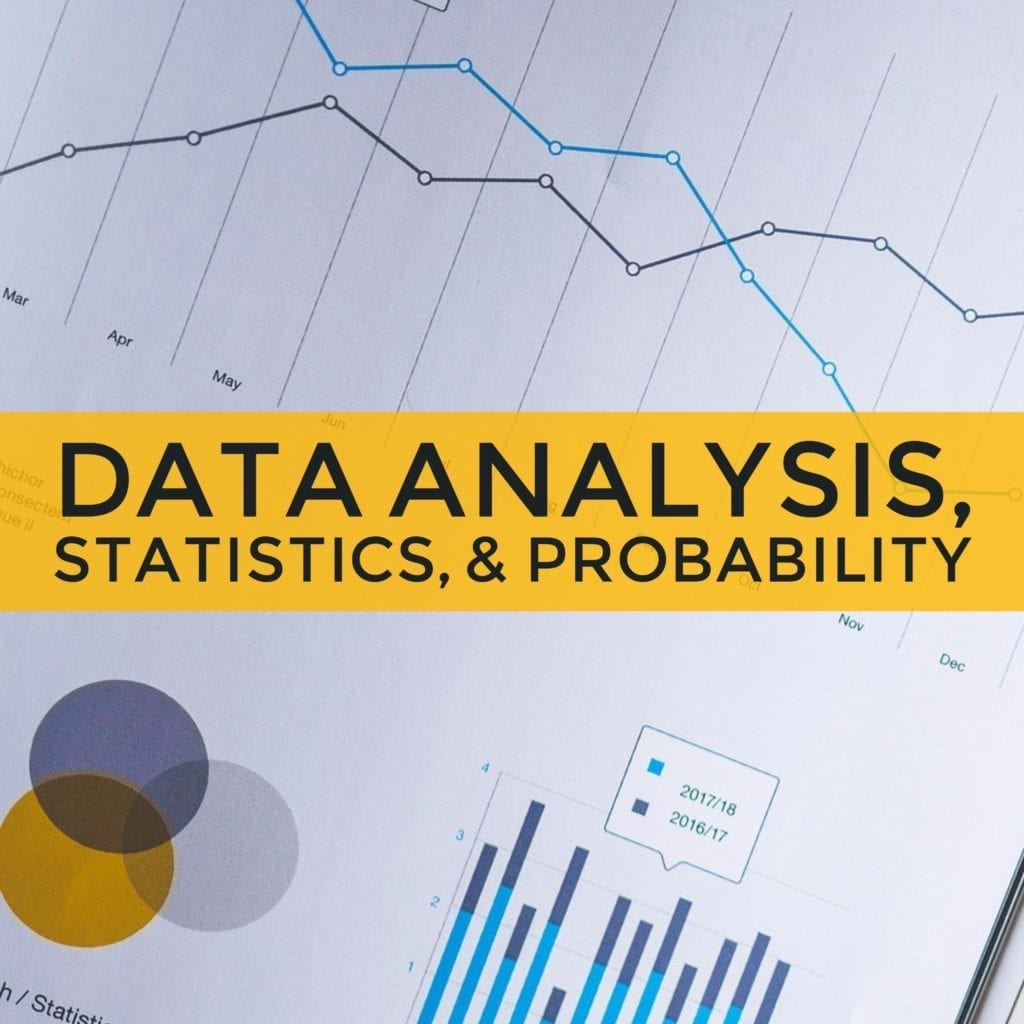# Data Analysis, Statistics, and Probability

Data Analysis, Statistics, and Probability are mathematical processes that help solve real-world problems. Probability helps predict the likelihood that an event will happen. To help predict the likelihood of events, people use statistical information and data. In mathematics, the probability is a branch that studies the results or consequences of mathematical data.

On the other hand, Statistics is a branch of mathematics that helps you make sense of data.

Data can often be presented in graphs, tables, and charts. Analyzing the data in these forms will help in decision-making as well as problem-solving.

Some of the most important statistical calculations include computing the sample size and population; the mean, mode, and median; standard deviation; and variance.

For the GED test, you might be asked read graphs and charts and determine the probability of simple and compound events. You might also be asked to find the mean, median, mode and range of a data set.

The mean is the average of all the given numbers. To compute for the mean, you need to add all the values and divide the sum by the number of all the given values.

The median according to the GED formula sheet, is the middle value in an odd number of ordered values of a data set or the average between the two middle values in an even number of ordered values in a data set.

The mode is the value that is repeated most often in a given set of values.

The range is the difference between the highest and lowest numbers within the given set of values.

The simplest formula for finding the probability is to divide the number of ways an event can happen by the number of possible outcomes.

Here is a sample of a statistics and probability problems:

1. Find the mean, median, mode, and range for this set of values:

35, 12, 13, 16, 20, 20,  21, 33, 40, 16, 18, 20, 9

Mean: _____ ?

Median: _____ ?

Mode: ____ ?

Range: _____ ?

1. There are 20 pieces of balloons at a party. 15 are pink, 5 are yellow. What is the probability that a pink balloon gets chosen by a child?

For more lessons on probability and statistics, watch section 3 of our GED math video lessons and take our GED practice tests.